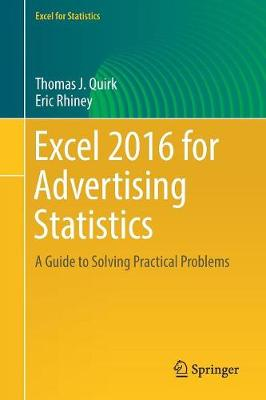Find your perfect holiday reading•# Excel 2016 for Advertising Statistics: A Guide to Solving Practical Problems - Excel for Statistics (Paperback)

(author), (author)
£45.99
Paperback 249 Pages / Published: 22/01/2018
• We can order this

Usually dispatched within 3 weeks

• This item has been added to your basket

This text is a step-by-step guide for students taking a first course in statistics for advertising and for advertising managers and practitioners who want to learn how to use Excel to solve practical statistics problems in in the workplace, whether or not they have taken a course in statistics.

Excel 2016 for Advertising Statistics explains statistical formulas and offers practical examples for how students can solve real-world advertising statistics problems. This book leaves detailed explanations of statistical theory to other statistics textbooks and focuses entirely on practical, real-world problem solving.

Each chapter briefly explains a topic and then demonstrates how to use Excel commands and formulas to solve specific advertising statistics problems. This book gives practice in using Excel in two different ways: (1) writing formulas (e.g., confidence interval about the mean, one-group t-test, two-group t-test, correlation) and (2) using Excel's drop-down formula menus (e.g., simple linear regression, multiple correlation and multiple regression, and one-way ANOVA). Three practice problems are provided at the end of each chapter, along with their solutions in an Appendix. An additional Practice Test allows readers to test their understanding of each chapter by attempting to solve a specific practical advertising statistics problem using Excel; the solution to each of these problems is also given in an Appendix.

* Includes 167 illustrations in color
* Suitable for undergraduates or graduate students

Publisher: Springer International Publishing AG
ISBN: 9783319721033
Number of pages: 249
Weight: 567 g
Dimensions: 235 x 155 mm
Edition: 1st ed. 2017

## Reviews

Sign In To Write A Review

Please sign in to write a review

Your review has been submitted successfully.